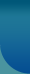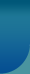Home >> Research Methods and Statistics >> Methods of Central Tendency of Averages

## Methods of Central Tendency of Averages

Condensation of data is necessary for a proper statistical analysis. A large number of big numbers are not only confusing to mind but also difficult to analyse.After a thorough scrutiny of collected data, classification which is a process of arranging data into different homogenous classes according to resemblances and similarities is carried out first.

Then of course tabulation of data is resorted to. The classification and tabulation of the collected data besides removing the complexity render condensation and comparison.

An average is defined as a value which should represent the whole mass of data. It is a typical or central value summarizing the whole data. It is also called a measure of central tendency for the reason that the individual values in the data show some tendency to centre about this average. It will be located in between the minimum and the maximum of the values in the data.

There are five types of average which are

1.Arithmatic Mean
2.Median
3.Mode
4.Geometric Mean and
5. Harmonic Mean

## Arithmetic Mean

The Arithmetic mean or simply the mean is the best known easily understood and most frequently used average in any statistical analysis. It is defined as the sum of all the values in the data.

## Median

Median is another widely known and frequently used average.It is defined as the most central or the middle most value of the data given in the form of an array. By an array, we mean an arrangement of the data either in ascending order or descending order of magnitude. In the case of ungrouped data one has to form an array first and then locate the middle most value which is the median. For ungrouped data the median is fixed by using,

Median = [n+1/2] the value in the array.

## Mode

The word mode seems to have been derived French 'a la mode' which means 'that which is in fashion'. It is defined as the value in the data which occurs most frequently. In other words, it is the most frequently occurring value in the data. For ungrouped data we form the array and then fix the mode as the value which occurs most frequently. If all the values are distinct from each other, mode cannot be fixed. For a frequency distribution with just one highest frequency such data are called unimodal or two highest frequencies [such data are called bimodal],mode is found by using the formula,

Mode = l + cf2/f1+f2
Where l is the lower limit of the model class, c is its class interval f1 is the frequency preceding the highest frequency and f2 is the frequency succeeding the highest frequency.

## Relative merits and demerits of Mean, Median and Mode

### Mean:

The mean is the most commonly and frequently used average. It is a simple average, understandable even to a layman. It is based on all the values in a given data. It is easy to calculate and is basic to the calculation of further statistical measures of dispersion, correlation etc. Of all the averages, it is the most stable one. However it has some demerits. It gives undue weightages to extreme value. In other words it is greatly influenced by extreme values.Moreover; it cannot be calculated for data with open - ended classes at the extreme. It cannot be fixed graphically unlike the median or the mode. It is the most useful average of analysis when the analysis is made with full reference to the nature of individual values of the data.Inspite of a few shortcomings; it is the most satisfactory average.

### Median:

The median is another well-known and widely used average. It is well-defined formula and is easily understood. It is advantageously used as a representative value of such factors or qualities which cannot be measured. Unlike the mean, median can be located graphically. It is also possible to find the median for data with open ended classes at the extreme. It is amenable for further algebraic processes.However,it is an average, not based on all the values of the given data. It is not as stable as the mean. It has only a limited use in practice.

### Mode:

It is a useful measure of central tendency, as a representative of the majority of values in the data. It is a practical average, easily understood by even laymen. Its calculations are not difficult. It can be ascertained even for data with open-ended classes at the extreme. It can be located by graphical means using a frequency curve. The mode is not based on all the values in the data. It become less useful when the data distribution is not uni-model.Of all the averages, it is the most unstable average.© 2023 Sociology Guide.Com